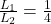## “On earth, you have a pendulum of length L that oscillates with period T. Your friend lives on a planet where the acceleration of gravity is

Question

“On earth, you have a pendulum of length L that oscillates with period T. Your friend lives on a planet where the acceleration of gravity is four times as big as it is on the earth. What should be the length of a pendulum on your friend s planet so that it also oscillates with the same period T

in progress 0
6 months 2021-08-22T05:32:30+00:00 2 Answers 1 views 0

## Answers ( )

4L

Explanation:

Data provided in the question according to the question is as follows

Length = L

Gravity = G

For friend

Length = ?

Growth = 4G

Moreover,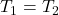Based on the above information ,

Now we have to apply the simple pendulum formula which is shown below: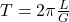Now equates these equations in both sides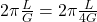So after solving this, the length of the pendulum is 4L

the length of a pendulum on your friend s planet should be 4 times than that on earth

Explanation:

We know that time period of simple pendulum is given by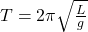L= length of pendulum

g= acceleration due to gravity

therefore, Let T_1 and T_2 be the time period of the earth and other planet respectively.ATQ

T_1=T_2=T,   g_2=4g_1

Putting the values in above equation and solving we get